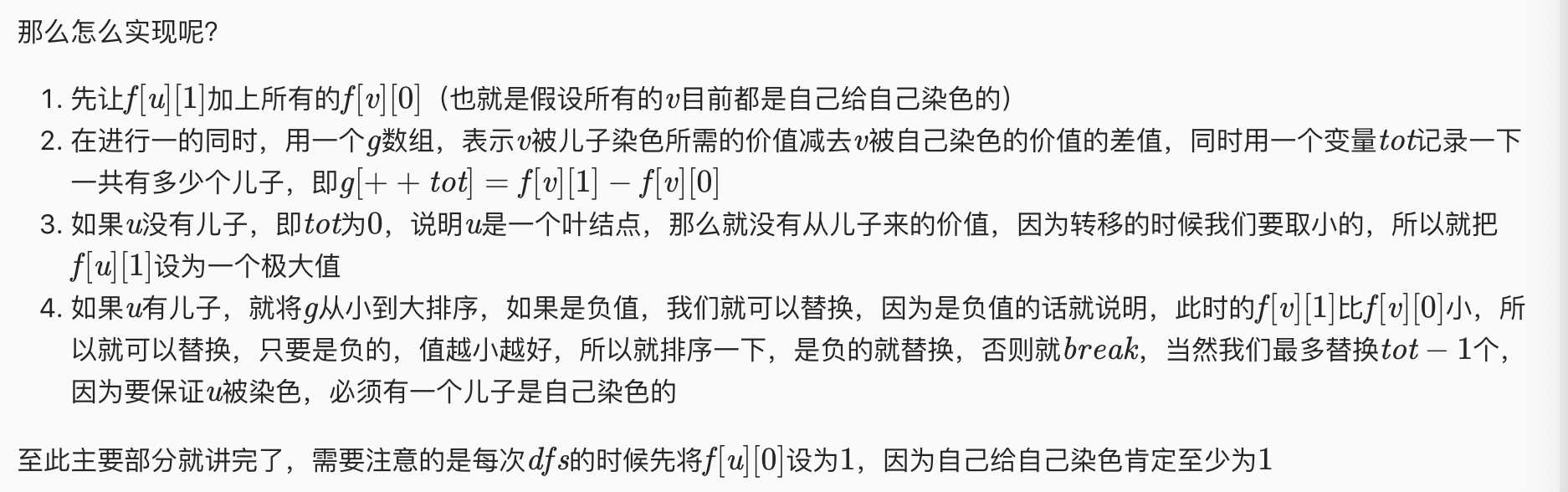# P2899 [USACO08JAN]手机网络Cell Phone Network

f[i] 代表被自己染色      f[i] 代表被父亲染色       f[i] 代表被儿子染色

#### 1自己被自己染色

f[u] += min(f[v],f[v],f[v] )

#### 2被自己的父亲结点染色

f[u] += min(f[v],f[v] )

#### 3被自己的儿子结点染色#include <iostream>
#include <algorithm>
#include <string>
#include <string.h>
#include <vector>
#include <map>
#include <stack>
#include <set>
#include <queue>
#include <math.h>
#include <cstdio>
#include <iomanip>
#include <time.h>
#include <bitset>
#include <cmath>

#define LL long long
#define INF 0x3f3f3f3f
#define ls nod<<1
#define rs (nod<<1)+1

const double eps = 1e-10;
const int maxn = 1e5 + 10;
const LL mod = 1e9 + 7;

int sgn(double a){return a < -eps ? -1 : a < eps ? 0 : 1;}
using namespace std;

struct edge {
int v,nxt;
}e[maxn << 1];

int cnt;
int f[maxn];

inline void add_edge(int u,int v) {
e[++cnt].v = v;
}

void dfs(int u,int fa) {
f[u] = 1;
int tot = 0;
int g[maxn];
for (int i = head[u];~i;i = e[i].nxt) {
int v = e[i].v;
if (v == fa)
continue;
dfs(v,u);
f[u] += min(f[v],min(f[v],f[v]));
f[u] += min(f[v],f[v]);
f[u] += f[v];
g[++tot] = f[v] - f[v];
}
if (!tot)
f[u] = INF;
else {
sort(g+1,g+1+tot);
for (int i = 1;i < tot;i++) {
if (g[i] < 0)
f[u] += g[i];
else
break;
}
}
}

int main() {
cnt = 0;
int n;
cin >> n;
for (int i = 1;i < n;i++) {
int u,v;
cin >> u >> v;
}
dfs(1,0);
cout << min(f,f) << endl;
return 0;
}

P2899 [USACO08JAN]手机网络Cell Phone Network

(0)
(0)